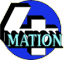# Free Maths Resources

As part of a new graphing package we are developing we have created some PDF files of various styles of graph paper which are now available to download.

• Printable graph paper - 1cm squares
• graph1cm.pdf (PDF file) - an A4 sheet of 1cm squares.
• graph1cm2.pdf (PDF file) - an A4 sheet of 1cm squares with 2 subdivisions.
• graph1cm3.pdf (PDF file) - an A4 sheet of 1cm squares with 3 subdivisions.
• graph1cm4.pdf (PDF file) - an A4 sheet of 1cm squares with 4 subdivisions.
• graph1cm5.pdf (PDF file) - an A4 sheet of 1cm squares with 5 subdivisions.
• graph1cm10.pdf (PDF file) - an A4 sheet of 1cm squares with 10 subdivisions.
• Printable graph paper - 2cm squares
• graph2cm.pdf (PDF file) - an A4 sheet of 2cm squares.
• graph2cm2.pdf (PDF file) - an A4 sheet of 2cm squares with 2 subdivisions.
• graph2cm3.pdf (PDF file) - an A4 sheet of 2cm squares with 3 subdivisions.
• graph2cm4.pdf (PDF file) - an A4 sheet of 2cm squares with 4 subdivisions.
• graph2cm5.pdf (PDF file) - an A4 sheet of 2cm squares with 5 subdivisions.
• graph2cm10.pdf (PDF file) - an A4 sheet of 2cm squares with 10 subdivisions.
• Printable graph paper - 4cm squares
• graph4cm.pdf (PDF file) - an A4 sheet of 4cm squares.
• graph4cm2.pdf (PDF file) - an A4 sheet of 4cm squares with 2 subdivisions.
• graph4cm3.pdf (PDF file) - an A4 sheet of 4cm squares with 3 subdivisions.
• graph4cm4.pdf (PDF file) - an A4 sheet of 4cm squares with 4 subdivisions.
• graph4cm5.pdf (PDF file) - an A4 sheet of 4cm squares with 5 subdivisions.
• graph4cm10.pdf (PDF file) - an A4 sheet of 4cm squares with 10 subdivisions.